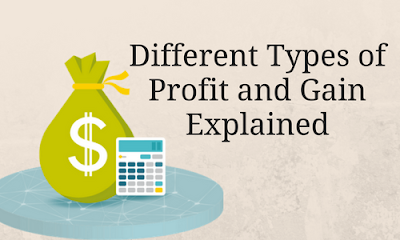# Different Types of Profit and GainA profit and gain both sound similar but have different meanings in the language of finance.
The main difference between profit and gain is that profit is the cumulative income for a certain period of time while the gain is a financial benefit which is derived from the arrangement of an asset above its net book value or market value.

## Profit

• In financial language, the word profit means the amount of revenue gained from a business activity exceeds the expenses, costs and taxes needed to sustain the activity.
• Profit is also known as the bottom line or net earnings.
• Profit is the real earnings of a business which indicates the financial position of the company as well.
• Profit is the positive earnings remained for a company after all costs and expenses have been subtracted from the total sales.
• Profit is all about what left out after subtracting expenses from the revenue.

### Types of Earnings

There are two types of earnings included in the profit which is as under
1) Direct earnings
It is the earnings which arrived from the main activities like selling of goods and services.

2) Indirect earnings
It is the earnings which came from the other activities like dividend from the shares, interest on property etc.

### Types of Profit

1) Gross profit
Gross profit is the fund from which trading expenses are deducted it means deduct the cost of goods from the revenue and what is left out is a gross profit.
Gross profit is calculated with the help of gross profit margin which shows the strength of the company like higher the gross profit margin, higher the efficiency of the business.

Formula:
Gross profit margin = Gross profit *100/ revenue

2) Operating Profit
Operating profit is arrived by adding net operating profit to the gross profit and then from it deducts the net operating expenses and what is left out is operating profit.
Higher operating profit shows the higher efficiency of the business and vice a versa.
Operating profit is measured by the operating profit margin formula.

Formula:
Operating Profit Margin = Operating Profit * 100 / Revenue

3) Net Profit
Net profit is the definitive profit amount which arrived after subtracting interest and taxes from the operating profit.
If there is a high level of net profit it will increase the value of the shares.

Formula
Net Profit Margin = Net Profit * 100 / Revenue

## Gain

• In a financial language, gain is the accretion in owner's equity which results from the other activities rather than day to day earnings from the repeated activity.
• The gain is indicated as a financial benefit which is derived not from the regular business activity but from the extra activity outside of a business like gain on sale of land and change in a stock’s market price.
• Gains are written in the income statement after the operating profit under the section of other revenues.
• For example: If a machinery is purchased at a cost of RS 10,000 and sold it at RS 12,000. it is called gain.

## Types of Gain

1) Realized Gain
Realized gain is the one which has occurred and realized soon.

For example:

A company has purchased a share of Rs 100 each and after some time the price of shares increase to Rs 125 each. So there is the gain and once it is sold in the market and it becomes a realized gain.

2) Unrealized Gain
Unrealized gain is the one which has occurred but it has been not realized yet.

For example:
A company has purchased a share of Rs 100 each and after some time the price of shares increase to Rs 125 each. So there is the gain but it is not sold that is why it is called an unrealized gain.